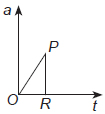# User Forum

Subject :NSO    Class : Class 9

A cyclist starts from centre O and reaches at R along the path OPR as shown in graph. What would you conclude from the velocity-time graph of the cyclist from the given graph?A Velocity changes linearly if acceleration is changing non-linearly.
B Velocity becomes zero if acceleration becomes zero.
C Velocity changes non-linearly if acceleration is changing linearly.
D Velocity becomes uniform if acceleration becomes infinite.

Give a nice sol pls